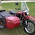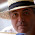## 10 August 2014

### Quiz 2: Find sum of all the numbers using STDIN.

Problem:
Ask user how many number he wants to add and then add them.

Sample Input:
Taken as <STDIN>

Sample Output:
Sum is xyz

Solution:

print "How many numbers you want to add\n";
my \$len = <STDIN>;
my \$tmp = 1;
my \$sum = 0;
while(\$tmp <= \$len)
{
print "Enter number \$tmp\n";
my \$var = <STDIN>   ;
\$sum = \$sum+\$var;
++\$tmp;
}
print "Sum is \$sum";

Tips:

• <STDIN> is used to take user input and save it in a variable.

#### 4 comments:

1.Two suggested changes: First, rather than make your user count the number of numbers in their list, just read values until you get a blank line. Second, add a \n to your result print stmt, so that the prompt doesn't print right after your output.

My version:
#! /usr/bin/perl

use strict;

#print "How many numbers you want to add\n";
#my \$len = ;
my \$tmp = 1;
my \$sum = 0;

print "Enter number \$tmp\n";
my \$var = ;
chomp \$var;

while(length(\$var) > 0)
{
\$sum = \$sum+\$var;

++\$tmp;
print "Enter number \$tmp\n";
\$var = ;
chomp \$var;
}
print "Sum is \$sum\n";

1.@robert: problem statement required count of numbers, so i coded this way

2.Also, what's wrong with

\$sum += \$var;

1.@Dave: nothing wrong, i just want to make it simple.